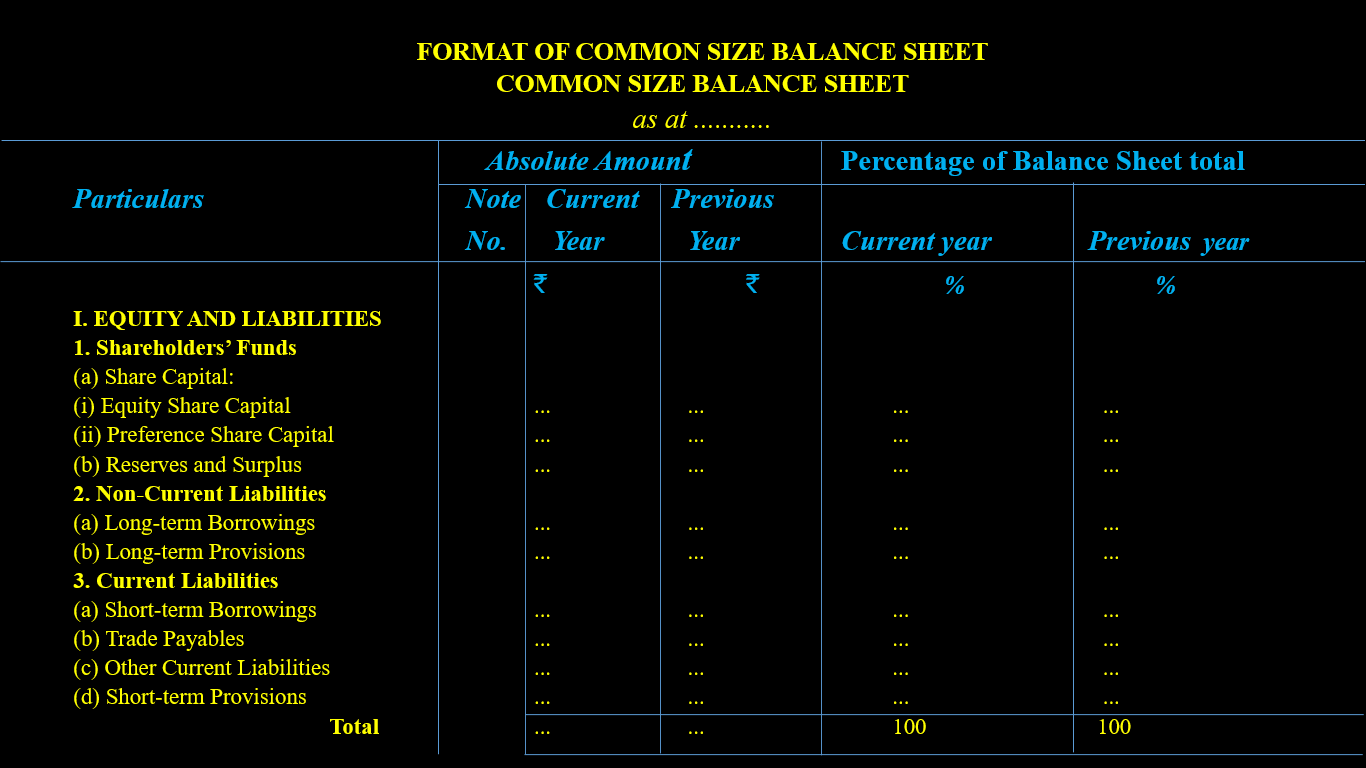# Common Size Statement

## Common Size Statement?

A common Size Statement is a statement that shows all items of the financial statement (Balance sheet & Statement of Profit and Loss) as a percentage on some common base.

Common Size Financial Statements are the statements in which amounts of the various items of financial statements are converted into percentages to a common base. The figures of these statements are shown as percentages of total assets, total liabilities, and total sales respectively.

In the words of Kohler, “Commonsize Statements are accounting statements expressed in percentage of some base rather than rupees”.

## Key Points of Common Size Statement:

• Common size statement is a financial tool.
• Common size statement is also known as vertical analysis or as component percentage or 100 per cent statements.
• Common size statement may be prepared for intra-firm and inter-firm comparison.
• Common size statement shows each item of the financial statement in absolute value.
• Common size statement shows each item of the financial statement as a percentage on some common base.
• Common size statement may be prepared for Balance Sheet as well as Statement of Profit and Loss.

## Types of Common Size Statements:

1. Common Size Balance Sheet
2. Common Size Statement of Profit and Loss

### 1. Common Size Balance Sheet:

Common Size Balance Sheet is a statement in which every item of assets, equity and liabilities is expressed as a percentage to the total of all assets or to the total of Equity and Liabilities.
Total Assets or Total Equity & Liabilities are taken as Common base.

Common Size Balance Sheet to be prepared as a percentage of total assets and total liabilities.

### Objectives Common Size Balance Sheet:

1. To analyse the changes in individual items of balance sheet.
2. To see the trend of different items of assets, equity and liabilities.
3. To assess the financial soundness.
4. To understand financial strategy adopted by different enterprises.

Preparation of  Common Size Balance Sheet:
Common size balance sheet is prepared by taking the following steps:

1. First column:  In this column, items of Balance Sheet are written.
2. Second column: In this column, Note number is written.
3. Third column:In this column, absolute figures of different items (assets, equity and liabilities) of current year are written.
4. Fourth column: In this column, absolute figures of different items (assets, equity and liabilities) of previous year are written.
5. Fifth column: In this column,percentage of different items of current year assets, equity, and liabilities to total assets/liabilities of current year(taken as 100) are written.
6. Sixth column: In this column, percentage of different items of previous year assets, equity, and liabilities to total assets/liabilities of previous year (taken as 100) are written.

### Format Of Common Size Balance SheetAlso read: Liquid Ratio Or Quick Ratio Or Acid Test Ratio

### 2. Common Size Statement of Profit and Loss:

Common Size Income Statement or Statement of Profit & Loss is a statement in which every item of Statement of Profit and Loss is expressed as a percentage of the amount of Revenue from Operations.
Sales (Revenue from operations) is taken as base.

Common size Statement of Profit and Loss to be prepared as a percentage of revenue from operations.

### Objectives Common Size Statement of Profit and Loss:

1. To analyse the changes in individual items of Statement of Profit and Loss.
2. To see the trend of different items of Statement of Profit and Loss.
3. To establish a relationship between individual items of Statement of Profit & Loss and revenue from operations.
4. To assess the efficiency.

Preparation of Statement of Profit and Loss :
Statement of Profit & Loss is prepared by taking the following steps:

1. First column: In this column, items of Statement of Profit & Loss are written.
2. Second column: In this column, Note number is written.
3. Third column: In this column, absolute figures of different items (Income and Expenses) of current year are written.
4. Fourth column: In this column, absolute figures of different items (Income and Expenses) of previous year are written.
5. Fifth column: In this column, percentage of different items of current year Income and Expenses to revenue from operations of current year(taken as 100) are written.
6. Sixth column: In this column, percentage of different items of Income and Expenses to revenue from operations of previous year(taken as 100) are written.

### Format Of Statement of Profit and Loss :• In common size statement of Profit & Loss take revenue from operation as common base not the total revenue.
•  In common size Balance Sheet take Balance Sheet total amount either total assets or total liabilities as common base.